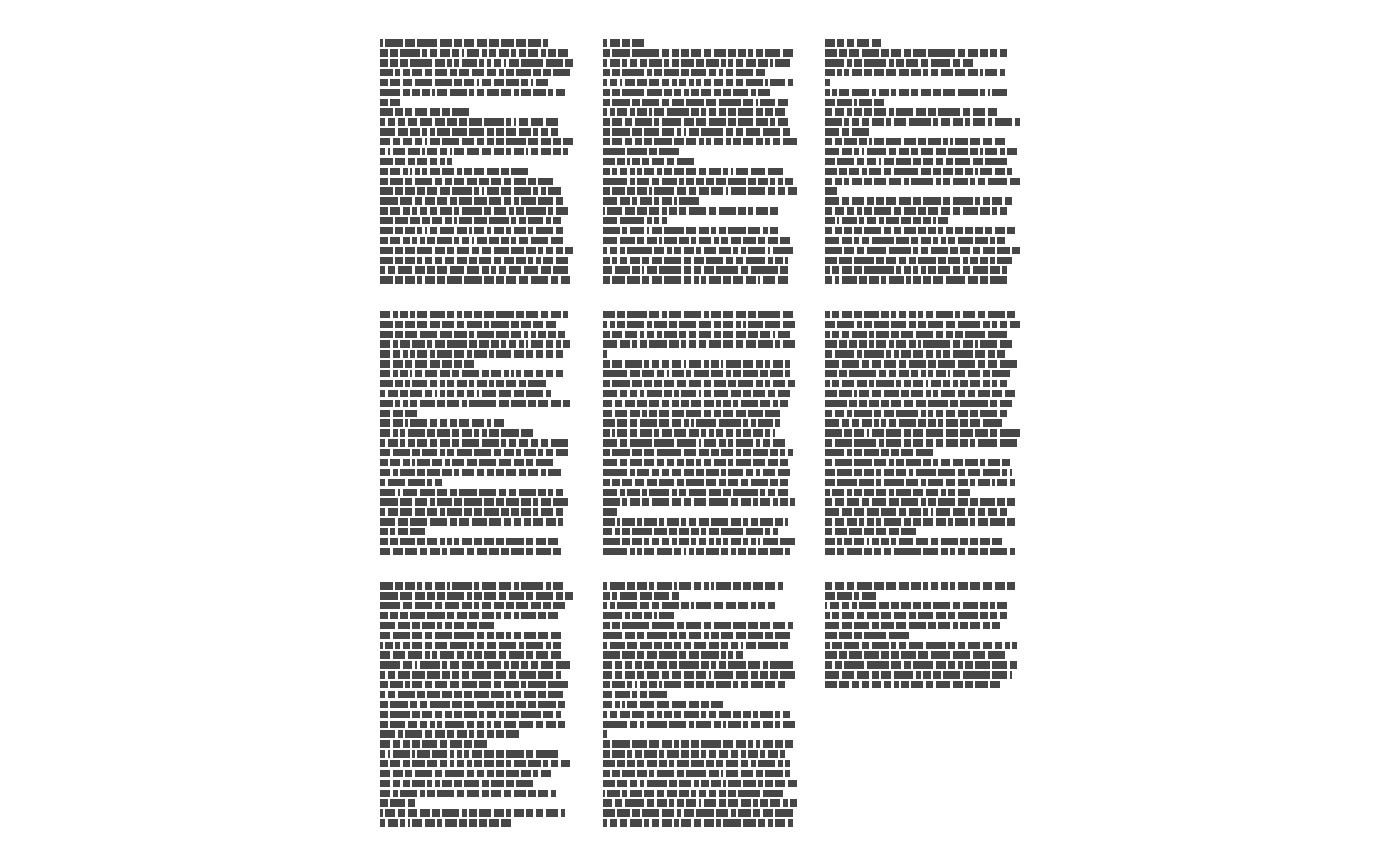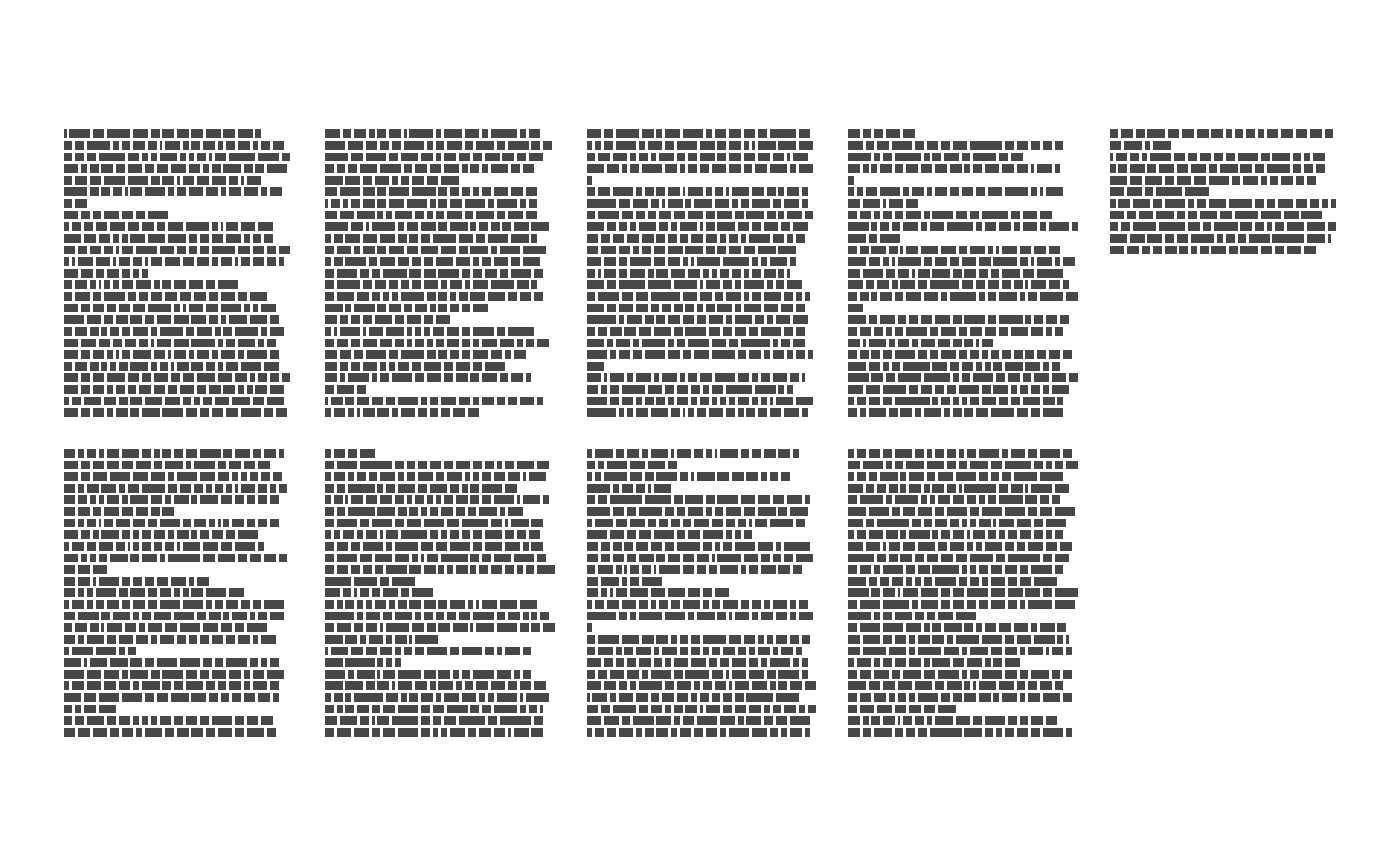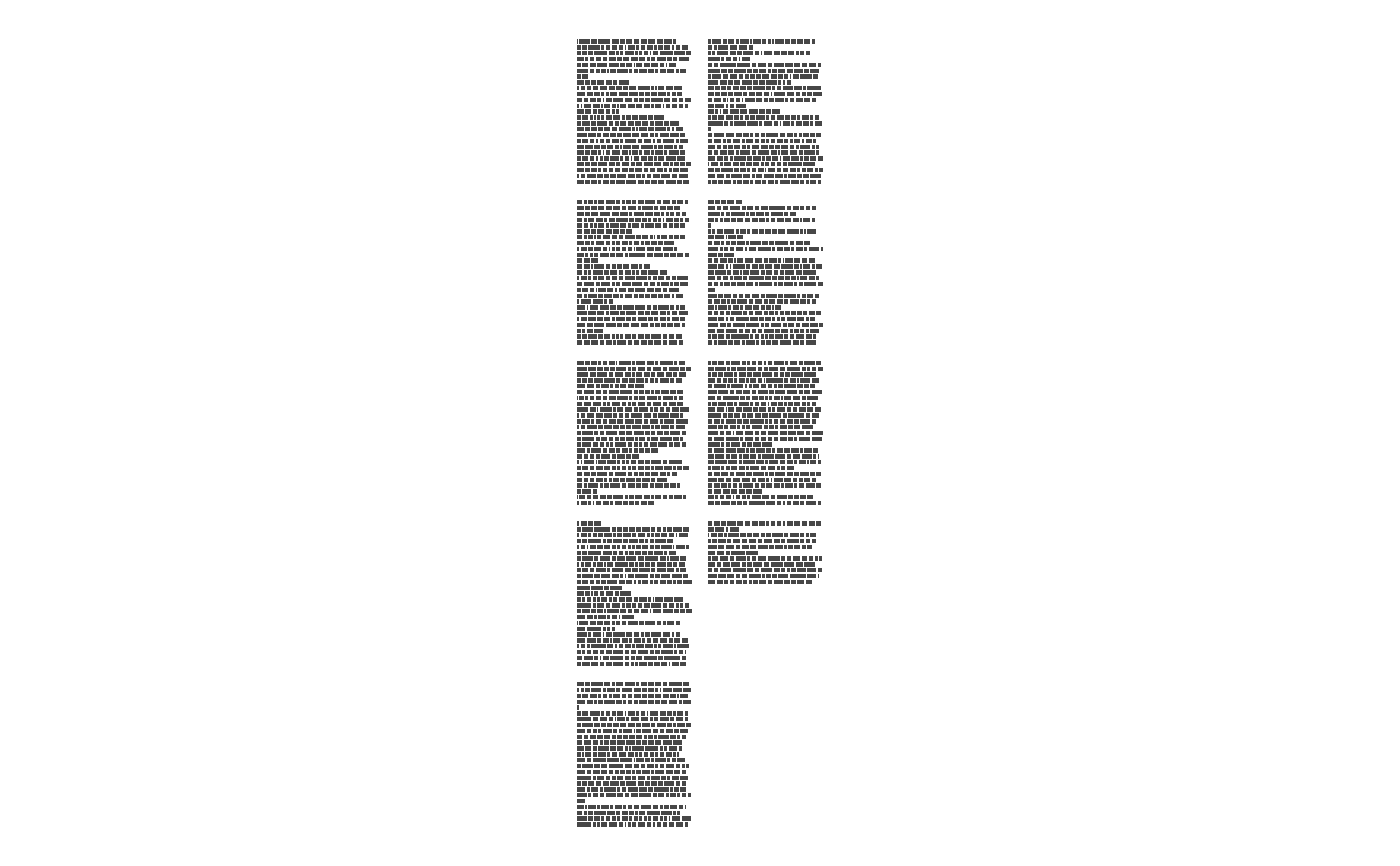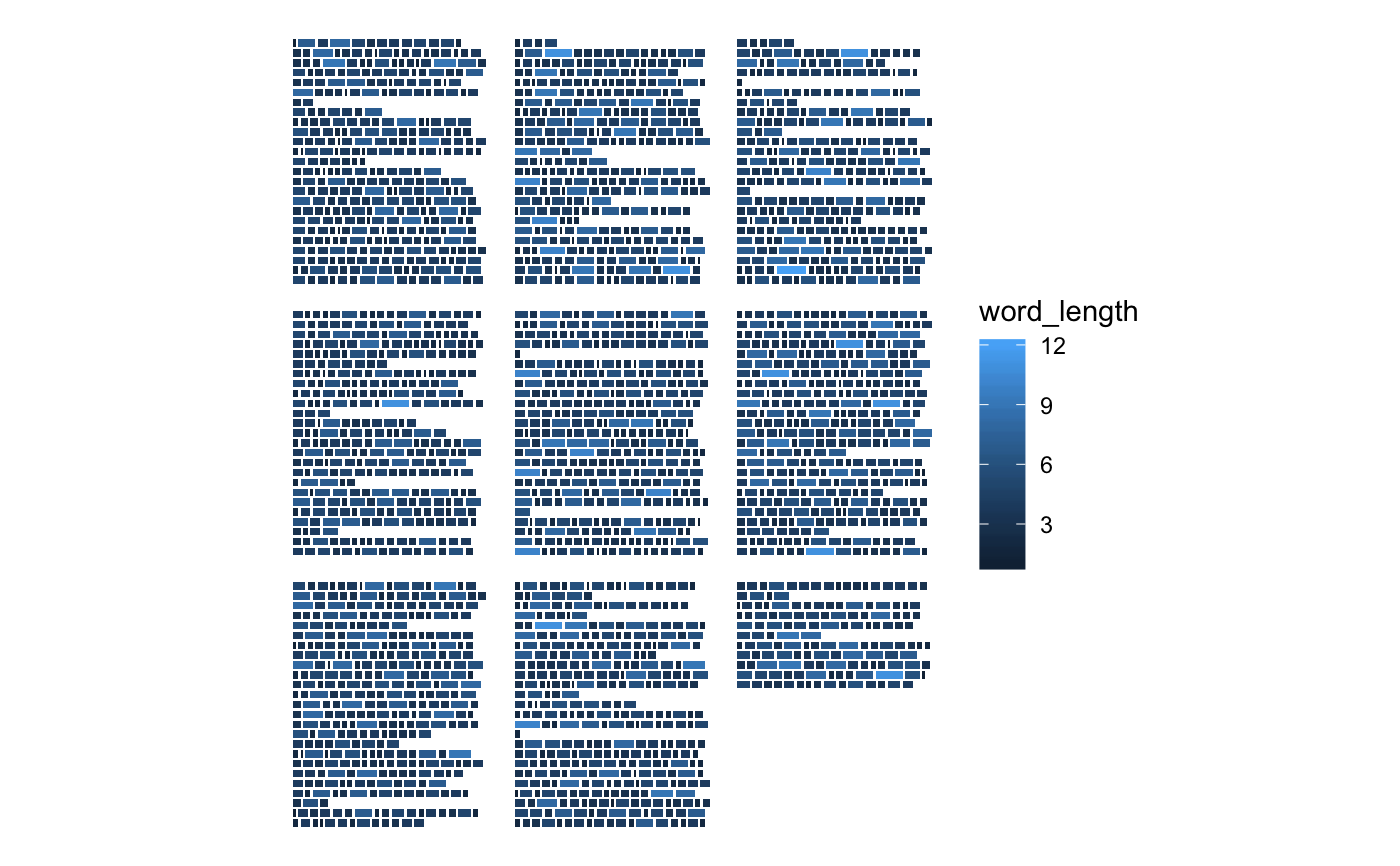This function can be used in combination with ggpage_plot to get the same result as ggpage_quick. However by splitting the data.frame construction and plotting we are able to do intermediate analysis which can be included in the visualization.

ggpage_build(book, lpp = 25, character_height = 3,
vertical_space = 1, x_space_pages = 10, y_space_pages = 10,
nrow = NULL, ncol = NULL, bycol = TRUE, wtl = NULL,
para.fun = NULL, page.col = NULL, align = "left", line.max = 80,
...)

## Arguments

book Character or data.frame. Can either have each element be a separate line or having each element being separate words. Numeric. Lines Per Page. Number of lines allocated for each page. Numeric. Relative size of the height of each letter compared to its width. Numeric. Distance between each lines vertically. Numeric. Distance between pages along the x-axis. Numeric. Distance between pages along the y-axis. Numeric. Number of rows of pages, if omitted defaults to square layout. Numeric. Number of columns of pages, if omitted defaults to square layout. Logical. If TRUE (the default) the matrix is filled by columns, otherwise the matrix is filled by rows. logical. If TRUE will convert single word vector into a vector with full lines. (defaults to FALSE). Function that generates random numbers to determine number of word in each paragraph. column to split the pages by. Type of line alignment. Must be one of "left", "right" or "both". Maximal number of characters per line. Defaults to 80. Extra arguments.

## Value

data.frame containing the following columns:

• word: Character. The words of the text.

• page: Integer. Page number.

• line: Integer. Line number within the page.

• xmin: Numeric. Border of rectangle, used by ggpage_plot do not alter.

• xmax: Numeric. Border of rectangle, used by ggpage_plot do not alter.

• ymin: Numeric. Border of rectangle, used by ggpage_plot do not alter.

• ymax: Numeric. Border of rectangle, used by ggpage_plot do not alter.

## Details

The text MUST be presented in a column named text.

## Examples

library(dplyr)#>
#> Attaching package: ‘dplyr’#> The following object is masked from ‘package:testthat’:
#>
#>     matches#> The following objects are masked from ‘package:stats’:
#>
#>     filter, lag#> The following objects are masked from ‘package:base’:
#>
#>     intersect, setdiff, setequal, unionlibrary(stringr)
library(ggplot2)
library(tidytext)
library(ggpage)
# build and plot
## data.frame with full lines
ggpage_build(tinderbox) %>%
ggpage_plot()## vector with full lines
ggpage_build(book = tinderbox %>%
pull(text)) %>%
ggpage_plot()## data.frame with single words
ggpage_build(tinderbox) %>%
unnest_tokens(text, word) %>%
ggpage_plot()## vector with single words
ggpage_build(tinderbox %>%
unnest_tokens(text, text) %>%
pull(text)) %>%
ggpage_plot()# nrow and ncol
ggpage_build(tinderbox, nrow = 2) %>%
ggpage_plot()ggpage_build(tinderbox, ncol = 2) %>%
ggpage_plot()# Include analysis within
ggpage_build(tinderbox) %>%
mutate(word_length = str_length(word)) %>%
ggpage_plot(aes(fill = word_length))